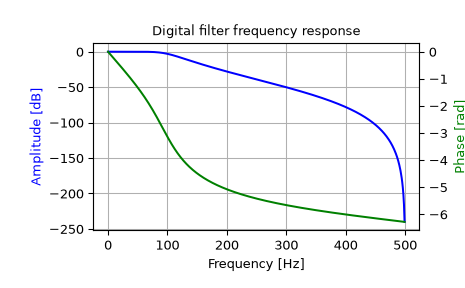# scipy.signal.freqz_zpk¶

scipy.signal.freqz_zpk(z, p, k, worN=512, whole=False, fs=6.283185307179586)[source]

Compute the frequency response of a digital filter in ZPK form.

Given the Zeros, Poles and Gain of a digital filter, compute its frequency response:

$$H(z)=k \prod_i (z - Z[i]) / \prod_j (z - P[j])$$

where $$k$$ is the gain, $$Z$$ are the zeros and $$P$$ are the poles.

Parameters
zarray_like

Zeroes of a linear filter

parray_like

Poles of a linear filter

kscalar

Gain of a linear filter

worN{None, int, array_like}, optional

If a single integer, then compute at that many frequencies (default is N=512).

If an array_like, compute the response at the frequencies given. These are in the same units as fs.

wholebool, optional

Normally, frequencies are computed from 0 to the Nyquist frequency, fs/2 (upper-half of unit-circle). If whole is True, compute frequencies from 0 to fs. Ignored if w is array_like.

fsfloat, optional

The sampling frequency of the digital system. Defaults to 2*pi radians/sample (so w is from 0 to pi).

New in version 1.2.0.

Returns
wndarray

The frequencies at which h was computed, in the same units as fs. By default, w is normalized to the range [0, pi) (radians/sample).

hndarray

The frequency response, as complex numbers.

freqs

Compute the frequency response of an analog filter in TF form

freqs_zpk

Compute the frequency response of an analog filter in ZPK form

freqz

Compute the frequency response of a digital filter in TF form

Notes

New in version 0.19.0.

Examples

Design a 4th-order digital Butterworth filter with cut-off of 100 Hz in a system with sample rate of 1000 Hz, and plot the frequency response:

>>> from scipy import signal
>>> z, p, k = signal.butter(4, 100, output='zpk', fs=1000)
>>> w, h = signal.freqz_zpk(z, p, k, fs=1000)

>>> import matplotlib.pyplot as plt
>>> fig = plt.figure()
>>> ax1 = fig.add_subplot(1, 1, 1)
>>> ax1.set_title('Digital filter frequency response')

>>> ax1.plot(w, 20 * np.log10(abs(h)), 'b')
>>> ax1.set_ylabel('Amplitude [dB]', color='b')
>>> ax1.set_xlabel('Frequency [Hz]')
>>> ax1.grid()

>>> ax2 = ax1.twinx()
>>> angles = np.unwrap(np.angle(h))
>>> ax2.plot(w, angles, 'g')

>>> plt.axis('tight')